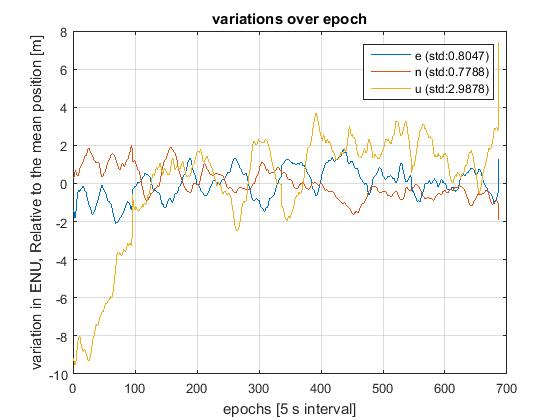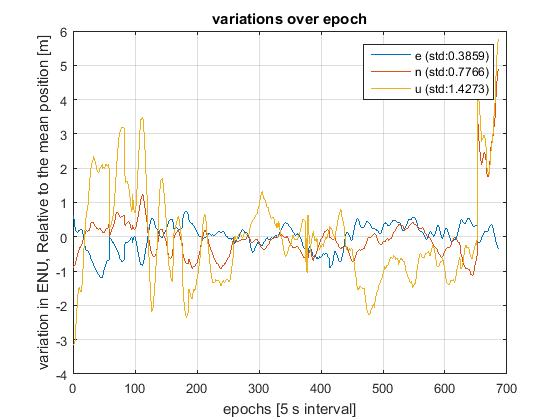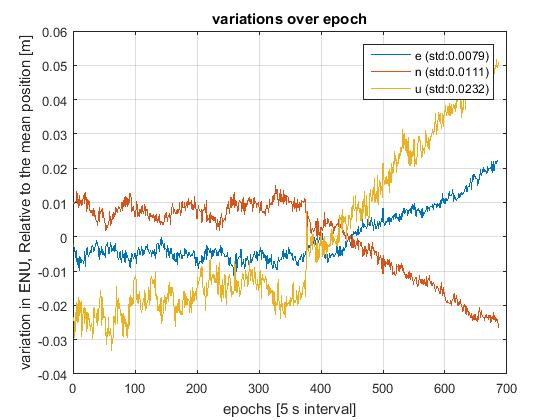# GPS几种基本定位模式原理概述及精度介绍

• 单点定位
• 伪距差分
• RTK

## 单点定位

${P}_{i}=p+c\ast dt+\mathrm{\Delta }trop+\mathrm{\Delta }ion+ϵ$

$p=\sqrt{\left({x}_{s}-{x}_{u}{\right)}^{2}+\left({y}_{s}-{y}_{u}{\right)}^{2}\left({z}_{s}-{z}_{u}{\right)}^{2}}$

P为伪距，可以从接收机的跟踪环路提取
xs,ys,zs为卫星位置，通过广播星历解算
c为光速dt为卫星时钟与接收机时钟之间的误差
trop和ion分别为对流层延迟和电离层延迟## 伪距差分## RTK

RTK（Real - time kinematic，实时动态）载波相位差分。关键算法包括单点定位，周跳探测和整周模糊度固定等，主要原理如下链接。